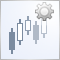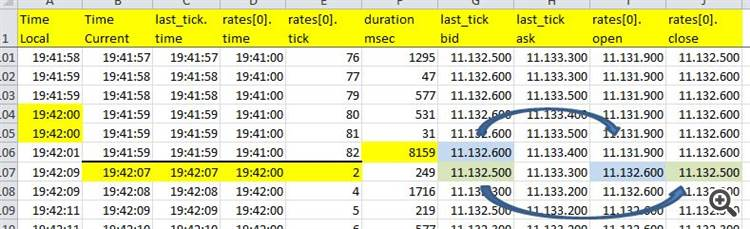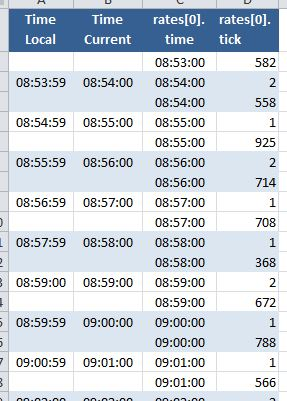# 表示一个新的酒吧

Mqlrates rates;

OnInit()
{
if(CopyRates(_Symbol,_Period,0,1,rates) < 1)
if(rates.tick_volume == 1)
{
... deal with new bar ...
}

OnInit()
{
if(BarsOld < Bars(_Symbol,_Period))
{
BarsOld = Bars(_Symbol,_Period);
... deal with new bar ...
}The "New Bar" Event Handler
• 2010.10.11
• Konstantin Gruzdev
• www.mql5.com
MQL5 programming language is capable of solving problems on a brand new level. Even those tasks, that already have such solutions, thanks to object oriented programming can rise to a higher level. In this article we take a specially simple example of checking new bar on a chart, that was transformed into rather powerful and versatile tool. What tool? Find out in this article.

TimeLocal (PC CLock), TimeCurrent(), 使用SymbolInfoTick()关于最后一个tick的一些信息，以及-使用CopyRates()-关于当前Bar的一些信息。1.TimeCurrent()是否总是等于刻度线的时间戳？

2.2.last_tick是否总是比新条形图的开盘时间早？

(待续)

TimeCurrent()是来自服务器的最后一个刻度的时间，这取决于你的代码是否来自你的图表符号。

//---  New Bar
bool   NewBar = false;
long   currPeriodSeconds;
double currPeriodProgress = 0;

int OnInit(void)  //-----------------------------------------------
{
currPeriodSeconds = PeriodSeconds();
return(INIT_SUCCEEDED);
}

void OnTick()  //--------------------------------------------------
{
if(MathMod(TimeCurrent(),currPeriodSeconds) < currPeriodProgress)
NewBar = true;
else NewBar = false;

currPeriodProgress = MathMod(TimeCurrent(),currPeriodSeconds);

//---  Just for testing
int    ExtHdlFile1=0;
MqlRates rates;

//---  New Bar
bool   NewBar = false;
long   currPeriodSeconds;
double currPeriodProgress = 0;

int OnInit(void) // -------------------------------------------------------
{
currPeriodSeconds = PeriodSeconds();

FileSeek (ExtHdlFile1,0,SEEK_END);
FileWrite(ExtHdlFile1,  "TimeLocal",
"TimeCurrent",
"rates.time",
"rates.tick");
return(INIT_SUCCEEDED);
}

void OnTick() // -----------------------------------------------------------
{
if(MathMod(TimeCurrent(),currPeriodSeconds) < currPeriodProgress)
NewBar = true;
else NewBar = false;

currPeriodProgress = MathMod(TimeCurrent(),currPeriodSeconds);

//--- lets check this

if(NewBar)
{
// last Time Stamp of old Bar
FileWrite(ExtHdlFile1, " ",
" ",
TimeToString(rates.time, TIME_MINUTES|TIME_SECONDS),
IntegerToString(rates.tick_volume));
// get the new bar
if(CopyRates(Symbol(),Period(),0,1,rates)!= 1) return;
// first Time Stamp of new Bar
FileWrite(ExtHdlFile1,TimeToString(TimeLocal(),TIME_MINUTES|TIME_SECONDS),
TimeToString(TimeCurrent(), TIME_MINUTES|TIME_SECONDS),
TimeToString(rates.time, TIME_MINUTES|TIME_SECONDS),
IntegerToString(rates.tick_volume));
}

if(CopyRates(Symbol(),Period(),0,1,rates)!= 1) return; // != clean code - just a test
}

void OnDeinit(const int reason)
{
FileClose(ExtHdlFile1);
return;
}

Willbur:// -----------------------------------------------------------------------
bool NewBar(void)
{
bool iNewBar = false;
static double currPeriodProgress = 0;

if(MathMod(TimeCurrent(),PeriodSeconds()) < currPeriodProgress) iNewBar = true;

currPeriodProgress = MathMod(TimeCurrent(),PeriodSeconds());

return(iNewBar);
}
// ------------------------------------------------------------------------
void OnTick()
{
if(NewBar())     PlaySound("tick.wav");

static datetime tlastbar=0;

datetime tnewbar=iTime(NULL,PERIOD_CURRENT,0)。

bool isnewbar=tnewbar!=tlastbar。

tlastbar=tnewbar。